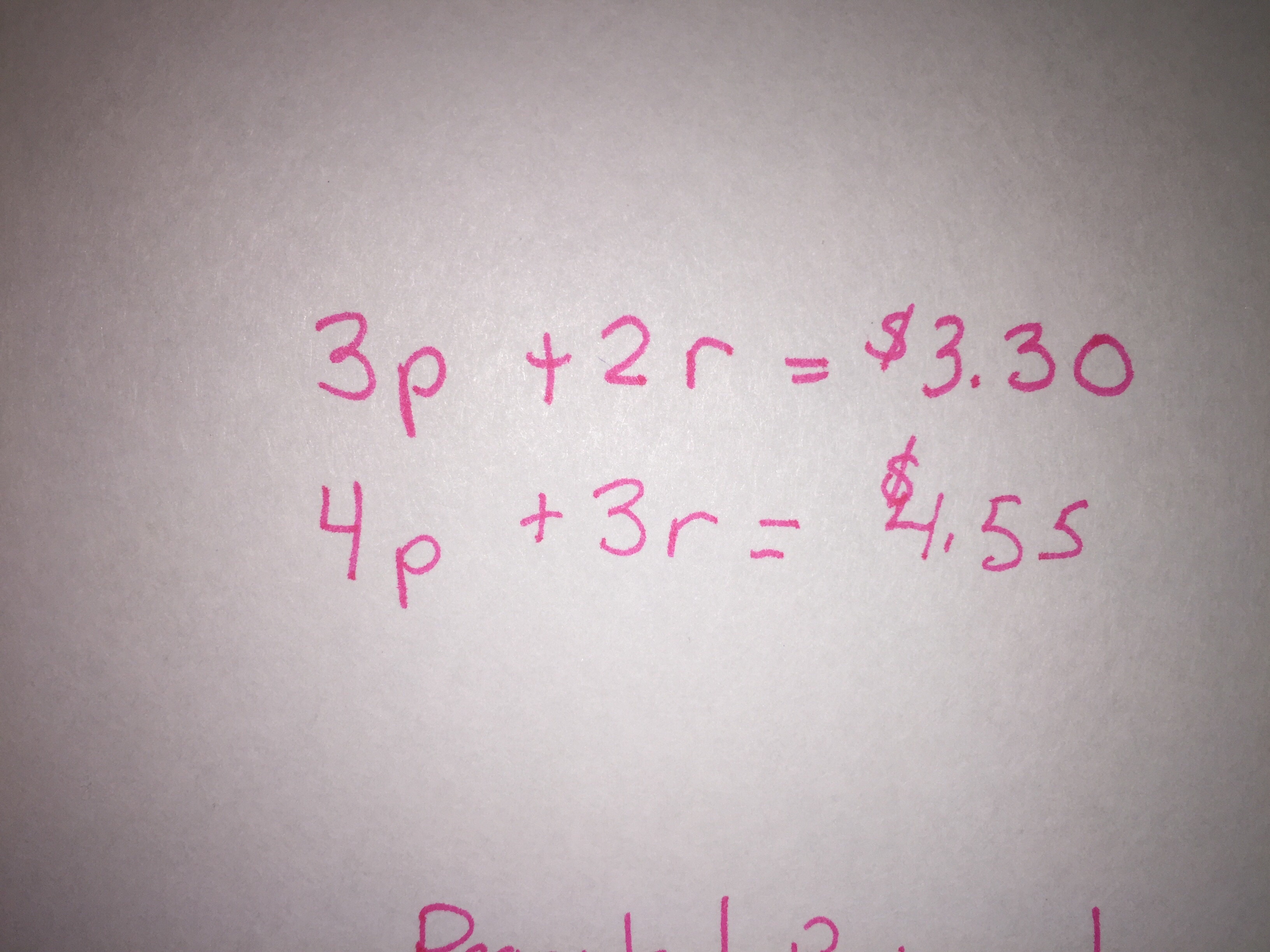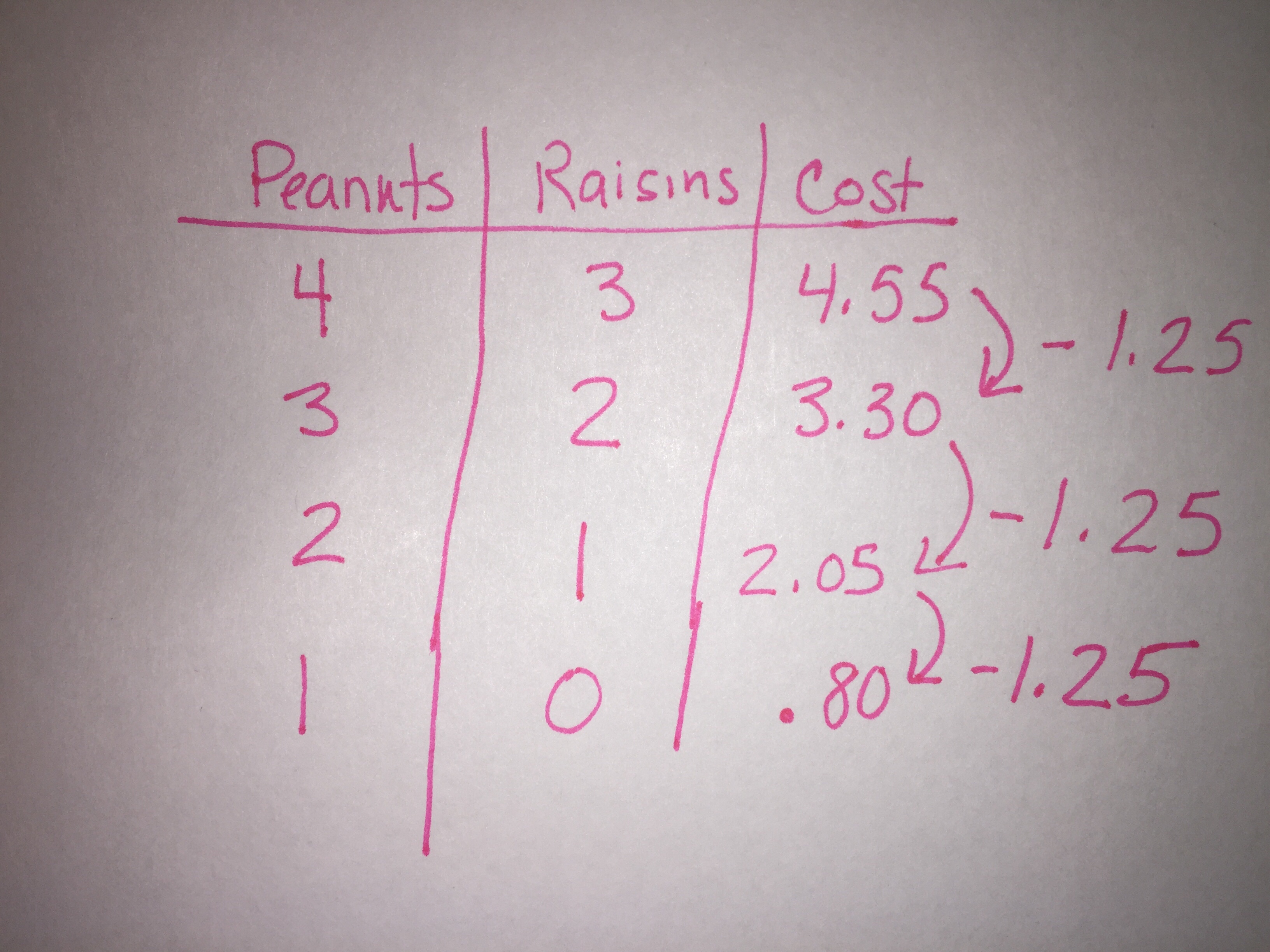# Grade 6 Math Week of 12/18/17

Over the past week, we’ve worked on strengthening our strategies for solving algebraic situations. Our scenarios have become less and less about direct exchange and more about looking at combinations with two distinct variables, and making sense of how to use what is known in the problem to determine the value of one of each variable. Once we know the value of one unit, it is easier to determine the value of any value for each variable.

A mixture of 3 cups of peanuts and 2 cups of raisins costs \$3.30.

A mixture of 4 cups of peanuts and 3 cups of raisins cost \$4.55.

What does it cost if you buy 5 cups of peanuts and 2 cups of raisins?This situation can be represented algebraically as it is in this photo where p=peanuts and r= raisins.Some students use a combination chart to see the relationship between the combinations and determine the unit rate for each item.A table can also show how students can use the known information to determine the relationship between variables. In this case, the cost of 4 cups of peanuts and 3 cups of raisins is \$4.55. When the amount of peanuts is reduced by 1 cup and the number of raisins is reduced by one cup the cost is \$1.25 less. If you continue the pattern, you quickly discover that 1 cup of peanuts and 0 cups of raisins is \$.80.Once you know the value of any one variable, you can replace the variable with that value and  determine the value (cost in this case) of the other variable.Ultimately, students will learn how to solve an algebraic situation using strategies such as the one above in 8th grade (Algebra 1). Students are not able to do this yet, and they are not expected to use this as a strategy. In 6th grade we focus on the relationships between the variables.

Understanding the relationships between the variables at this point is more important than knowing a procedure. Following steps does not mean that you understand why it works, and ultimately when we figure out the relationship mathematically, we gain stronger understanding. We will continue to practice solving for variables using our problem solving strategies and, sharing our strategies as a class to allow students multiple opportunities to see and try new strategies.

I also want to note, that students are still deliberately being given problems with numbers that are not complicated so that they can develop these relationships, and while students with strong number sense may immediately see relationships among numbers that lend themselves to quick ‘guess and check’ answers. Students are being encouraged to develop strategies that do not use ‘guess and check’ since relying only on that strategy in the future will slow them down.

This week we have already begun to look at situations with 3 variables and will add more strategies and tools to our bank. After break we’ll dust off the vacation cobwebs and jump back into our algebra work.

Have a happy, safe, and restful break!